# How to Run Python ScriptsLearn how you can execute a Python script from the command line, and also how you can provide command line arguments to your script.

Typically, every novice learns to write a Python script on the command line first, then moves to execute the script from the command line wherein the script is usually written in a text editor and is run from the command line.

Computers cannot understand code in the way humans write it and hence, you need an interpreter between the computer and the human written code. The job of the interpreter is to convert the code into a format that computers can then understand and process.

The interpreter processes the code in the following ways:

• Processes the Python script in a sequence

• Compiles the code into a byte code format which is a lower-level language understood by the computers.

• Finally, a Python Virtual Machine (PVM) comes into the picture. The PVM is the runtime powerhouse of Python. It is a process that iterates over the instructions of your low-level bytecode code to run them one by one.

Like Scripts, you have a have something called Module which is a Python script imported and used in another Python script.

The Python script is saved with a .py extension which informs the computer that it is a Python program script. Unlike Windows, the Unix based operating systems such as Linux and Mac come with pre-installed Python. Also, the way Python scripts are run in Windows and Unix operating systems differ.

Note: For all users, especially Windows OS users, it is highly recommended that you install `Anaconda`, which can be downloaded from this website. You can follow along with the instructions given in this DataCamp tutorial, which are specific to Windows operating system.

To install `Python3` on a Windows operating system, please feel free to check out this link.

Command-line interpreter for Python can be accessed on the various operating systems in the following ways:

• Processes the Python script in a sequence

• Compiles the code into a byte code format which is a lower-level language understood by the computers.

• Finally, a Python Virtual Machine (PVM) comes into the picture. The PVM is the runtime powerhouse of Python. It is a process that iterates over the instructions of your low-level bytecode code to run them one by one.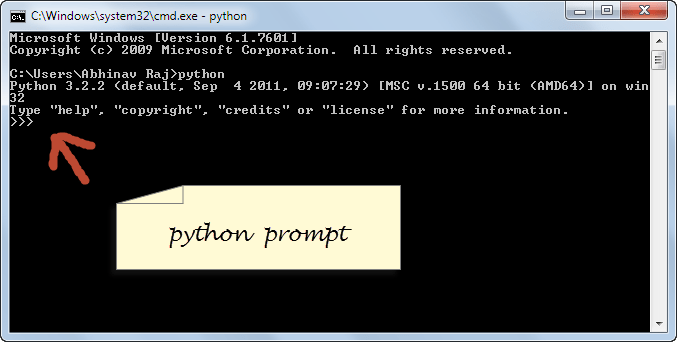(Source)

• Processes the Python script in a sequence

• Compiles the code into a byte code format which is a lower-level language understood by the computers.

• Finally, a Python Virtual Machine (PVM) comes into the picture. The PVM is the runtime powerhouse of Python. It is a process that iterates over the instructions of your low-level bytecode code to run them one by one.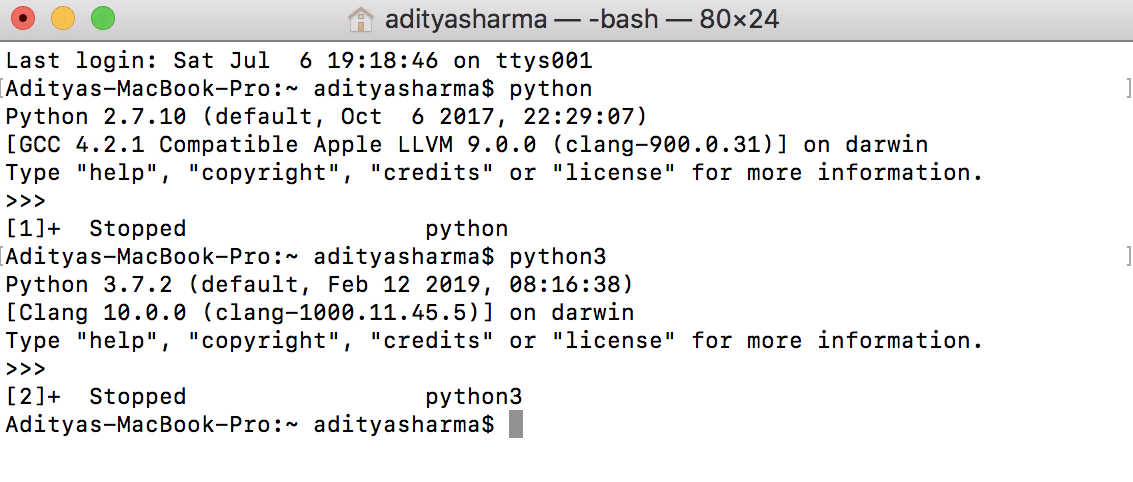• Processes the Python script in a sequence

• Compiles the code into a byte code format which is a lower-level language understood by the computers.

• Finally, a Python Virtual Machine (PVM) comes into the picture. The PVM is the runtime powerhouse of Python. It is a process that iterates over the instructions of your low-level bytecode code to run them one by one.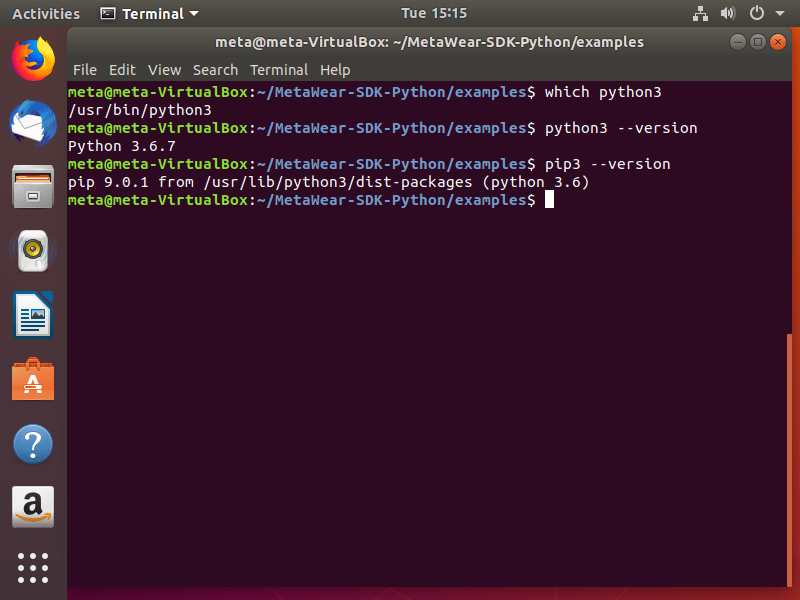(Source)

Note: In this tutorial, you will be learning using the Mac OS Terminal.

So without further ado, lets get started!

### Writing the Python Script in Terminal

Let's see how we can code and generate the output in the terminal itself. To accomplish this, first, you will type `python3`, which means you will be using Python3 version.

After which you can code typically as you would in a text editor or an IDE (integrated development environment), though you will not be getting the functionalities in the terminal as you would get with an IDE.

You will start with the evergreen `Hello, World!` example and let's see how the output looks like in the terminal.Isn't it nice how by just opening the terminal and typing Python3 you can code in Python? Let's try some more examples.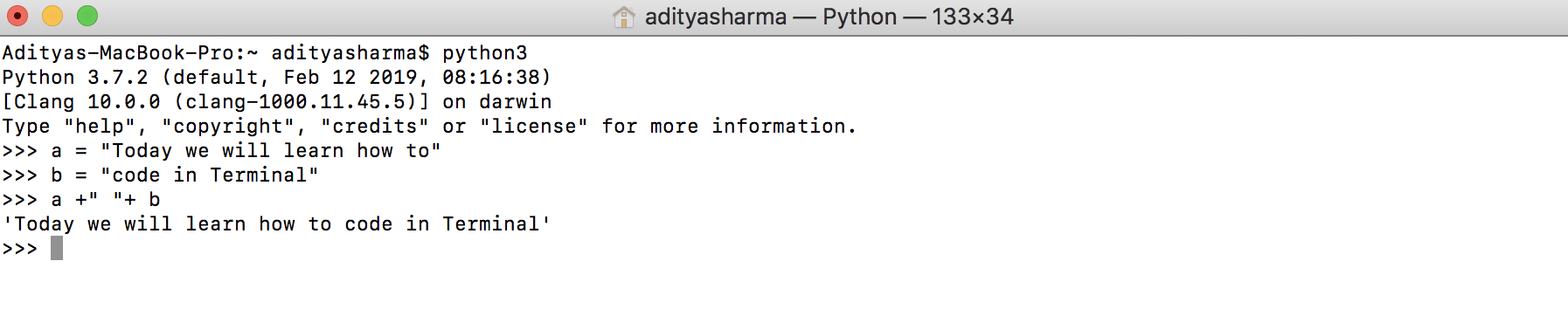One thing to notice in the above example is, without even typing the `print` statement you were able to get the output.

Let's complicate the code a bit and lets you use the `NumPy` (Numerical Python) library to create two arrays and apply a few mathematical operations on it. For a little background on numpy, it is a Python programming library which has the capability of dealing with large, multi-dimensional arrays and matrices, along with an extensive collection of high-level mathematical functions to operate on these arrays.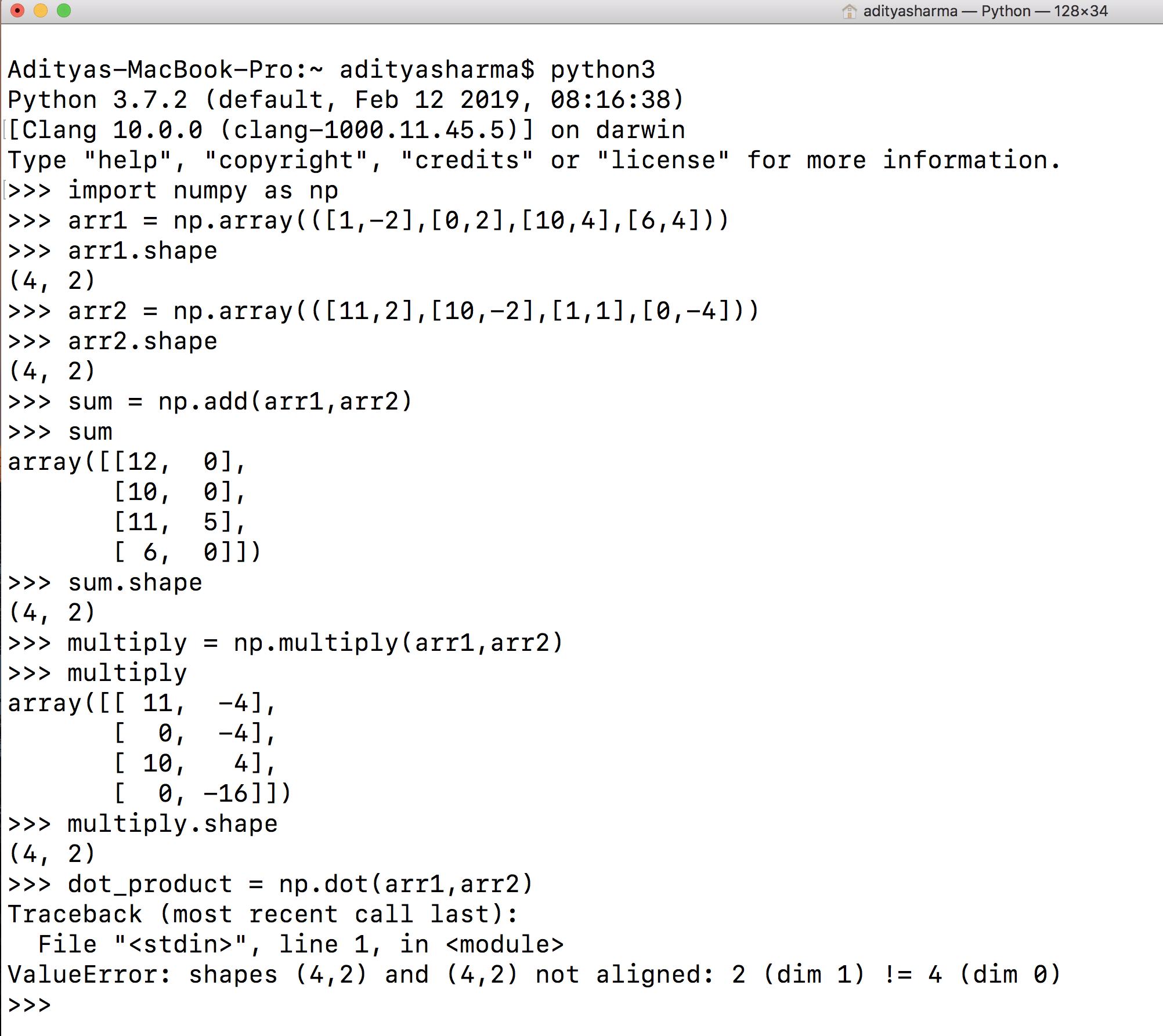You can observe from the above figure that using numpy; you were able to create, add, and multiply two numpy arrays within the terminal. One thing to note here is that the `multiply`function in numpy does an element-wise multiplication while `dot` function takes the dot product of two matrices. To accomplish the `np.dot` command, you need to make sure that the `columns` of the first matrix are equal to the `rows` of the second matrix and hence, the `value error`.

### Running the `**.py**` script from the Terminal

Running the Python script from the terminal is very simple, instead of writing the Python script in the terminal all you need to do is use a text editor like vim, emacs or notepad++ and save it with a `.py` extension. Then, open the terminal and go to the directory where the code resides and run the script with a keyword `python` followed by the script name.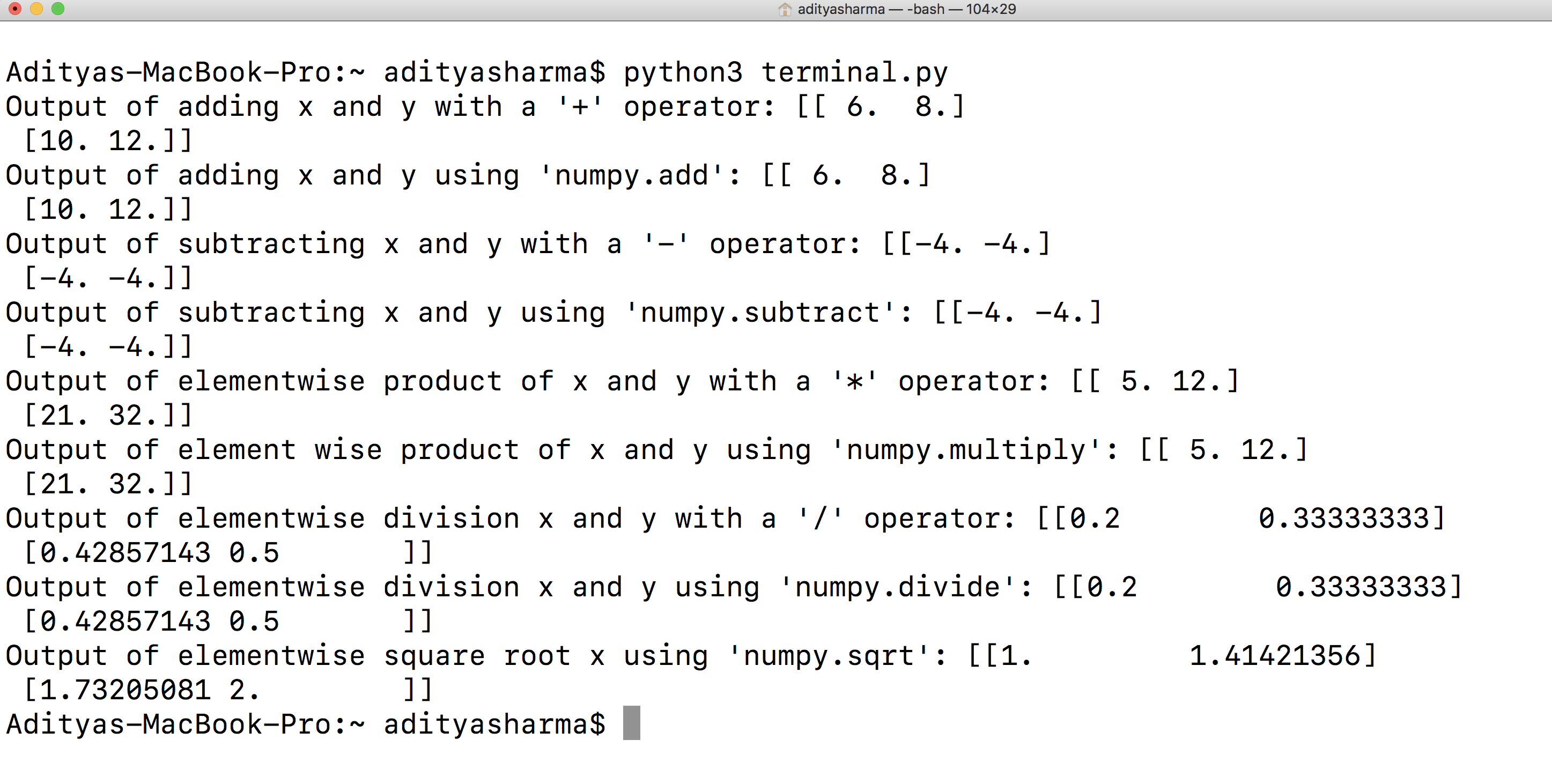To create the `terminal.py` file, use `vim` in the terminal with the program name as `vim terminal.py` and paste the below code in it. To save the code, press `esc` key followed by `wq!`. And finally, to run that script all you need to do is type `python3 terminal.py` and within less than a second you will see the output as shown above.

The following is the code which was used in the above example.

``````import numpy as np

x = np.array([[1,2],[3,4]], dtype=np.float64)
y = np.array([[5,6],[7,8]], dtype=np.float64)

# Elementwise sum; both produce the array
# [[ 6.0&nbsp; 8.0]
#&nbsp; [10.0 12.0]]
print("Output of adding x and y with a '+' operator:",x + y)

# Elementwise difference; both produce the array
# [[-4.0 -4.0]
#&nbsp; [-4.0 -4.0]]
print("Output of subtracting x and y with a '-' operator:",x - y)
print("Output of subtracting x and y using 'numpy.subtract':",np.subtract(x, y))

# Elementwise product; both produce the array
# [[ 5.0 12.0]
#&nbsp; [21.0 32.0]]
print("Output of elementwise product of x and y with a '*' operator:",x * y)
print("Output of element wise product of x and y using 'numpy.multiply':",np.multiply(x, y))

# Elementwise division; both produce the array
# [[ 0.2&nbsp; &nbsp; &nbsp; &nbsp; &nbsp;0.33333333]
#&nbsp; [ 0.42857143&nbsp; 0.5&nbsp; &nbsp; &nbsp; &nbsp;]]
print("Output of elementwise division x and y with a '/' operator:",x / y)
print("Output of elementwise division x and y using 'numpy.divide':",np.divide(x, y))

# Elementwise square root; produces the array
# [[ 1.&nbsp; &nbsp; &nbsp; &nbsp; &nbsp; 1.41421356]
#&nbsp; [ 1.73205081&nbsp; 2.&nbsp; &nbsp; &nbsp; &nbsp; ]]
print("Output of elementwise square root x using 'numpy.sqrt':",np.sqrt(x))
``````
``````Output of adding x and y with a '+' operator: [[ 6.&nbsp; 8.]
&nbsp;[10. 12.]]
&nbsp;[10. 12.]]
Output of subtracting x and y with a '-' operator: [[-4. -4.]
&nbsp;[-4. -4.]]
Output of subtracting x and y using 'numpy.subtract': [[-4. -4.]
&nbsp;[-4. -4.]]
Output of elementwise product of x and y with a '*' operator: [[ 5. 12.]
&nbsp;[21. 32.]]
Output of element wise product of x and y using 'numpy.multiply': [[ 5. 12.]
&nbsp;[21. 32.]]
Output of elementwise division x and y with a '/' operator: [[0.2&nbsp; &nbsp; &nbsp; &nbsp; 0.33333333]
&nbsp;[0.42857143 0.5&nbsp; &nbsp; &nbsp; &nbsp;]]
Output of elementwise division x and y using 'numpy.divide': [[0.2&nbsp; &nbsp; &nbsp; &nbsp; 0.33333333]
&nbsp;[0.42857143 0.5&nbsp; &nbsp; &nbsp; &nbsp;]]
Output of elementwise square root x using 'numpy.sqrt': [[1.&nbsp; &nbsp; &nbsp; &nbsp; &nbsp;1.41421356]
&nbsp;[1.73205081 2.&nbsp; &nbsp; &nbsp; &nbsp; ]]
``````

### Passing Command Line Arguments to Python Script

Command line arguments make life a lot easier, many times in production-ready code command line arguments are used. It makes the code output reproducibility efficient. For example, let's say your code has a lot of user inputs or files that are being read, then by using command line arguments you will not have to go into the code to do all of these changes in case you need to change the file name or a variable input.

To accomplish this, you will make use of the `sys` module, which is an in-built Python module. Within `sys`, you have `argv` which gives you the list of command-line arguments passed to the Python program. `sys.argv` reads the command line arguments as a list of items where the first item/element in that list can be accessed as `sys.argv` while first argument, i.e., `sys.argv`is always the name of the program as it was invoked.

Let's take a simple example and see how it works.

Note: The command line argument is read as a `string` by Python, so make sure to convert it as an `integer` in case you are dealing with numbers.

Much like the previous example, save the below lines of code in an editor as `command_line.py`and to run the code type `python3 command_line.py 10` where 10 is the command line argument.

``````import sys
num = sys.argv
for i in range(int(num)):
print (i)
``````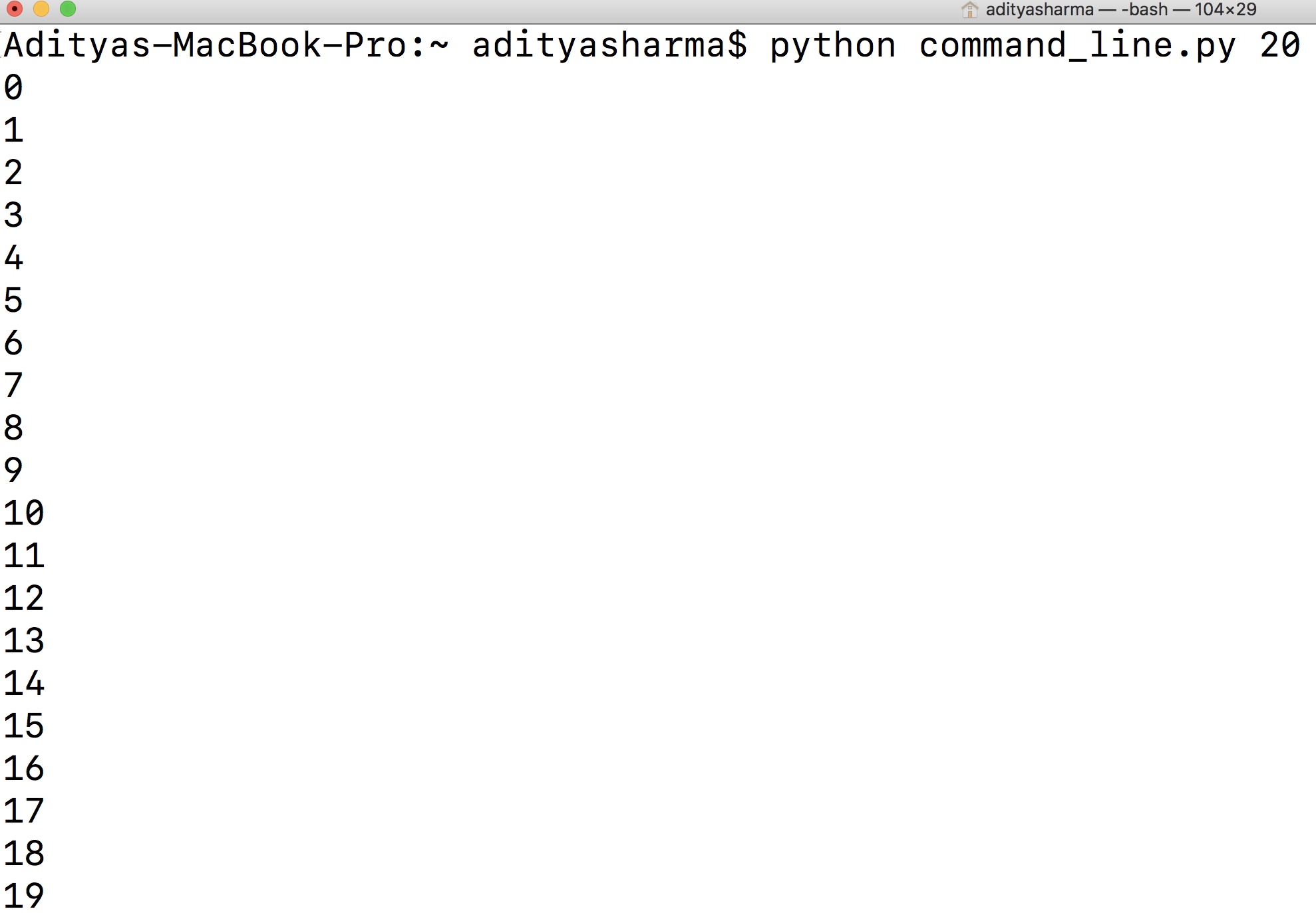#### What if you do not provide any command line argument?

You will get an error `list index out of range` which also reinforces that `sys.argv` reads as a list of items. To avoid such errors, you need `exceptional handling`, which is out of the scope for this tutorial.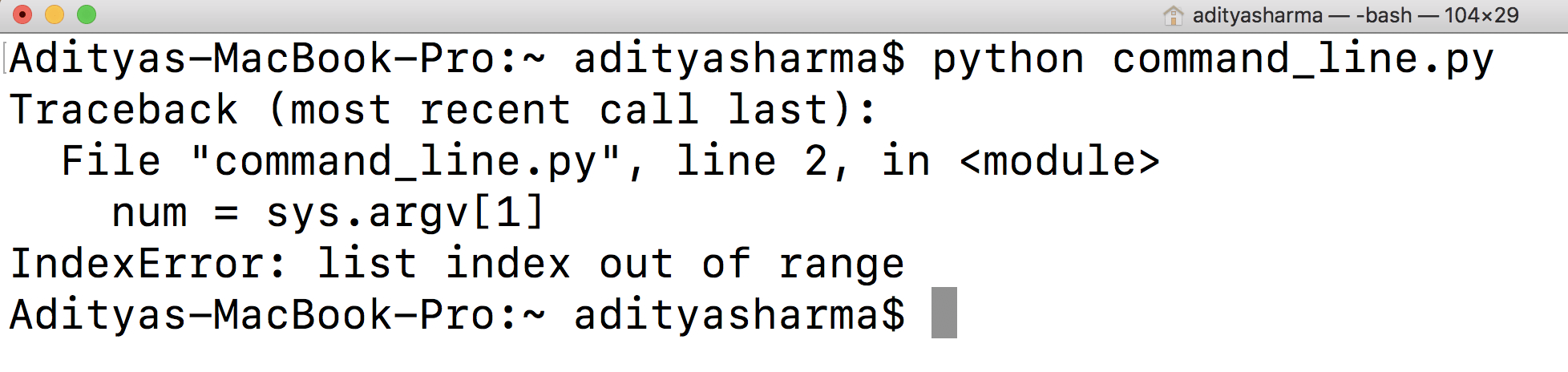### Writing the Output of Python Script to a File

Finally, as a bonus lets see how you can save the output of the Python script in a `txt` file with using the `>` key. Yes, one mere character, isn't that amazing?

You will first create a folder `cli` and move the `command_line.py` code in the `cli` folder. Then, you will check what all is there in that folder, to make sure there is no `txt` file already existing.

Then, you will type `python3 command_line.py 10 > output.txt` and finally check the content of the `cli` folder. Now, you should be able to see the `output.txt` file.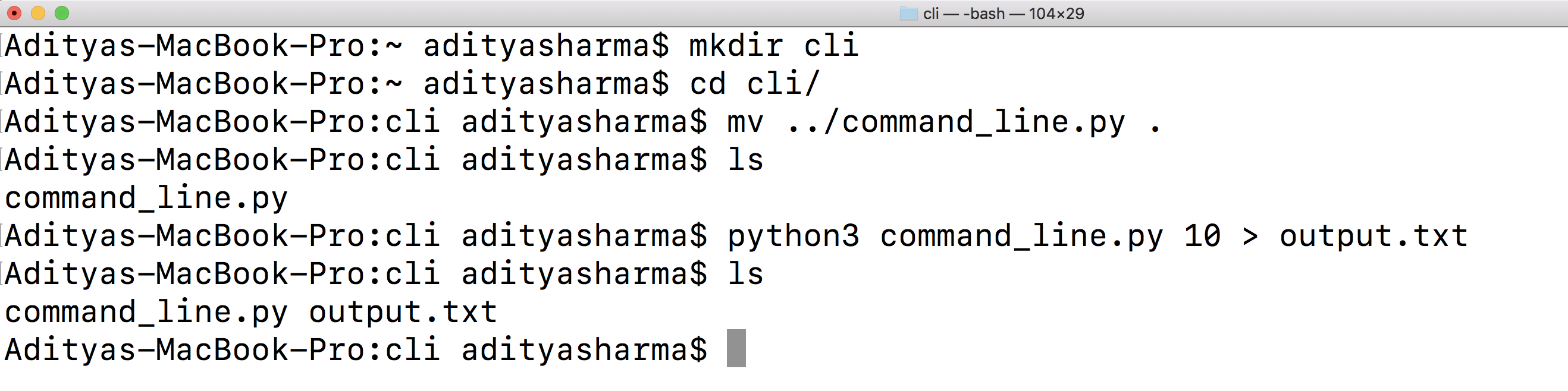Let's open and see the content of the `output.txt` file.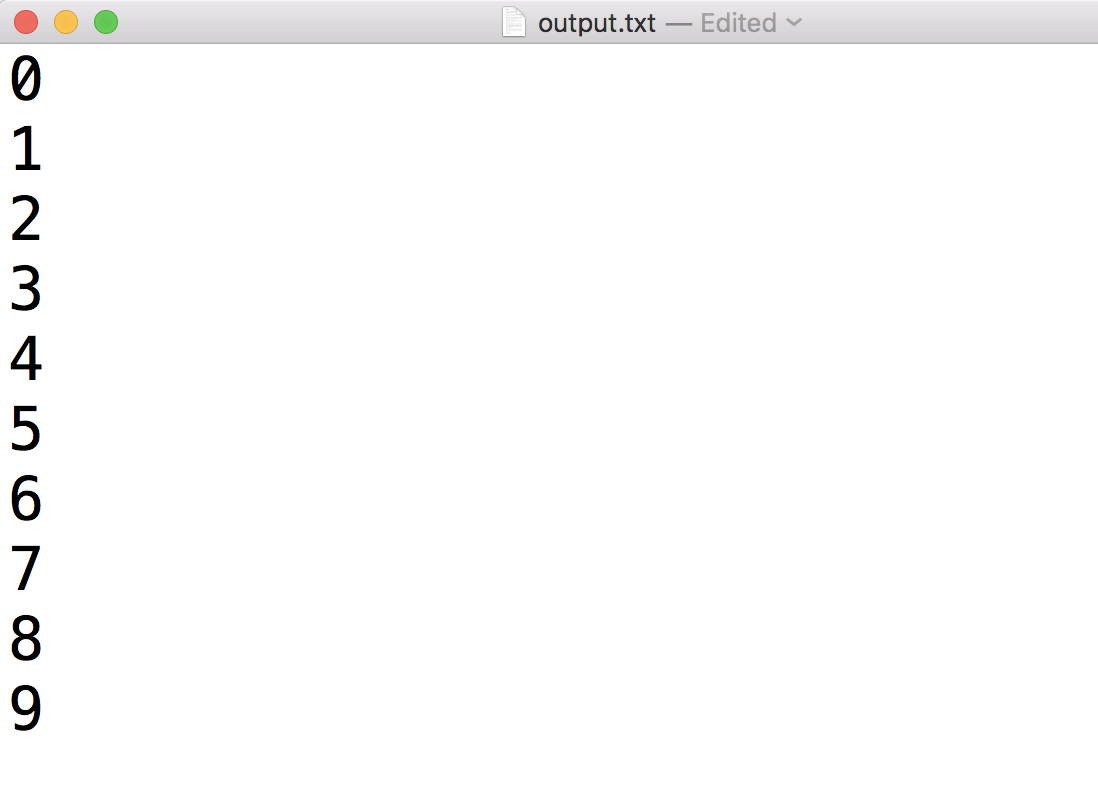Voila! From the above output, you can see that the `0-9` digits have now been written in a text file using just a `>` sign.

# Conclusion

Congratulations on finishing the tutorial.

There is still a lot to be explored like GUI based execution in Python, running Python scripts interactively, running scripts from a file manager or from an IDE. Specific to command line execution you might want to experiment on the Windows `command prompt` and see how it is different from a Unix based operating system.

## Basic Data Types in Python | Python Web Development For Beginners

In the programming world, Data types play an important role. Each Variable is stored in different data types and responsible for various functions. Python had two different objects, and They are mutable and immutable objects.

## How To Compare Tesla and Ford Company By Using Magic Methods in Python

Magic Methods are the special methods which gives us the ability to access built in syntactical features such as ‘<’, ‘>’, ‘==’, ‘+’ etc.. You must have worked with such methods without knowing them to be as magic methods. Magic methods can be identified with their names which start with __ and ends with __ like __init__, __call__, __str__ etc. These methods are also called Dunder Methods, because of their name starting and ending with Double Underscore (Dunder).

## Python Programming: A Beginner’s Guide

Python is an interpreted, high-level, powerful general-purpose programming language. You may ask, Python’s a snake right? and Why is this programming language named after it?

## Hire Python Developers

Are you looking for experienced, reliable, and qualified Python developers? If yes, you have reached the right place. At **[HourlyDeveloper.io](https://hourlydeveloper.io/ "HourlyDeveloper.io")**, our full-stack Python development services...

## Python any: How to Check If Element is Iterable or Not

Python any() function returns True if any element of an iterable is True otherwise any() function returns False. The syntax is any().